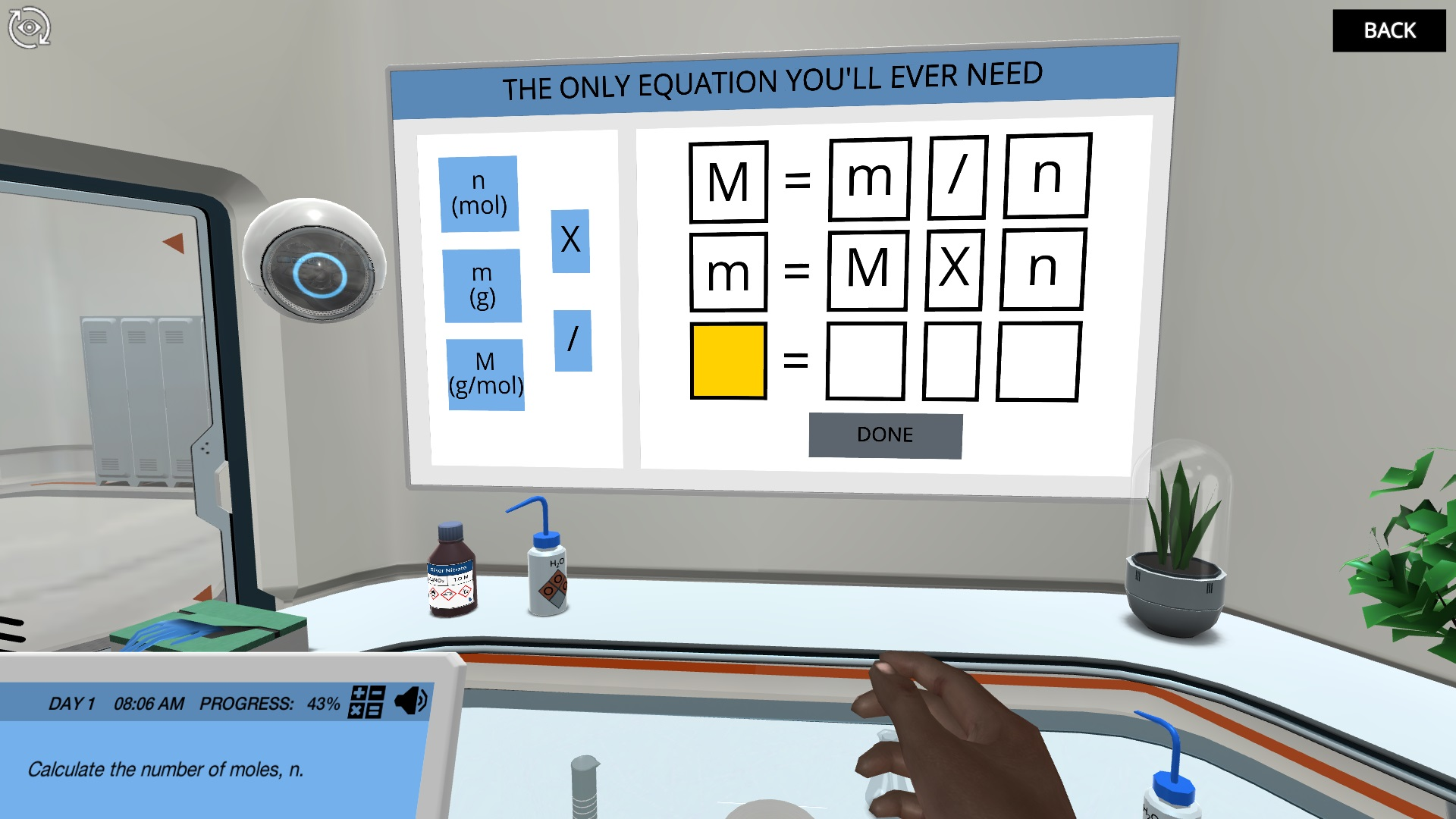Back to simulations

# Stoichiometric Calculations: Identify a compound using gravimetric analysis Virtual Lab

Learn about Avogadro’s number and the relationship between moles, mass and molecular weight as you use the technique of gravimetric analysis to identify an unknown compound.High SchoolUniversity / CollegeDid you know that there are more water molecules in a glass of water than there are sand grains in the Sahara desert? In this simulation, you will learn about the relationship between mass, molecular weight and the number of atoms or molecules, and you will understand the magnitude and importance of Avogadro’s number.

Identify an unknown compound

In order to identify a compound where the label has been partly destroyed, you must apply the technique of gravimetric analysis. To do so, you must first learn to understand the relationship between mass, moles and molecular weights and how to perform stoichiometric calculations from mass to mass via mole conversions.

Stoichiometric calculations with moles

You will perform a realistic gravimetric analysis with detailed instructions on what to do and why to do it in every step of the experiment. From balancing the equation to recognizing the stoichiometry of the reactants and finding out which equation to employ in the calculations, the theory behind the experiment is explained step-by-step in the order of the experiment.

What compound is it?

At the end of the simulation, you will have finalized all of the stoichiometric calculations, and the answer to the question should be clear… Can you see what compound it is?

#### Learning Objectives

At the end of this simulation, you will be able to:

• Explain the relationship between mass, molecular weight, and numbers of atoms or molecules and perform calculations deriving these quantities from one another

• Perform mass-to-mass stoichiometric calculations via conversions to mole

• Identify the limiting and excess reagents in a chemical reaction

• Calculate the theoretical, actual and percent reaction yield

• Define Avogadro’s number and describe the mole quantification of matter

#### Techniques in Lab

At the end of this simulation, you will be able to:

• Gravimetric analysis

## How do virtual labs work?

Engage students in science through interactive learning scenarios. Simulate experiments, train lab techniques, and teach theory through visual experiences that enhance long-term learning outcomes.300+ Web-based simulations that can be played on laptops, Chromebooks, and tablets/iPads without installing any softwareTeacher dashboard to automate grading and track student progressEmbedded quizzes to help students master science contentLibrary of learning resources, lab reports, videos, theory pages, graphics and more

### Get Started Now!

Try Labster with your students right away.Track student progressAssess with embedded quiz questionsInvite your students to play simulationsExplore over 300 Labster simulations